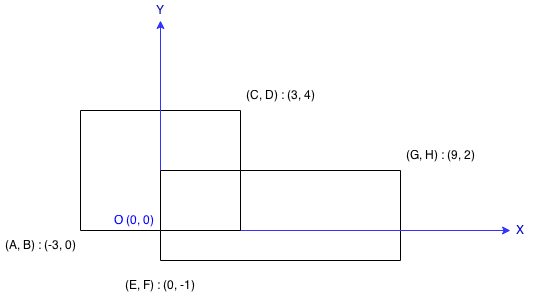# 223 Rectangle Area – Easy

### Problem:

Find the total area covered by two rectilinear rectangles in a 2D plane.

Each rectangle is defined by its bottom left corner and top right corner as shown in the figure.Assume that the total area is never beyond the maximum possible value of int.

### Thoughts:

There are two cases for this problem:

1. When two rectangle doesn’t have overlap, then the total area is the sum of two rectangle.
2. When two rectangle have overlap, then the total area is the sum of two rectangle minus the overlapped area.

### Solutions:

``````public class Solution {
public int computeArea(int A, int B, int C, int D, int E, int F, int G, int H) {
int total = (C - A) * (D - B) + (G - E) * (H - F);
if (C <= E || B >= H || A >= G || D<= F) {